# RS Aggarwal Solutions for Class 9 Chapter 3: Factorisation of Polynomials Exercise 3E

## RS Aggarwal Solutions for Class 9 Maths Exercise 3E PDF

The creative, talented and experienced set of experts in Mathematics at BYJU’S prepare the solutions for the exercises according to the RS Aggarwal textbook based on the students’ grasping capacity. The solutions are prepared in an interactive manner, thus making it more interesting for students while studying. Exercise 3E is the fifth exercise in the third chapter containing problems which are solved in brief to find the cube of a binomial using different methods. RS Aggarwal Solutions for Class 9 Maths Chapter 3 Factorisation of Polynomials Exercise 3E are provided here.

## RS Aggarwal Solutions for Class 9 Chapter 3: Factorisation of Polynomials Exercise 3E Download PDF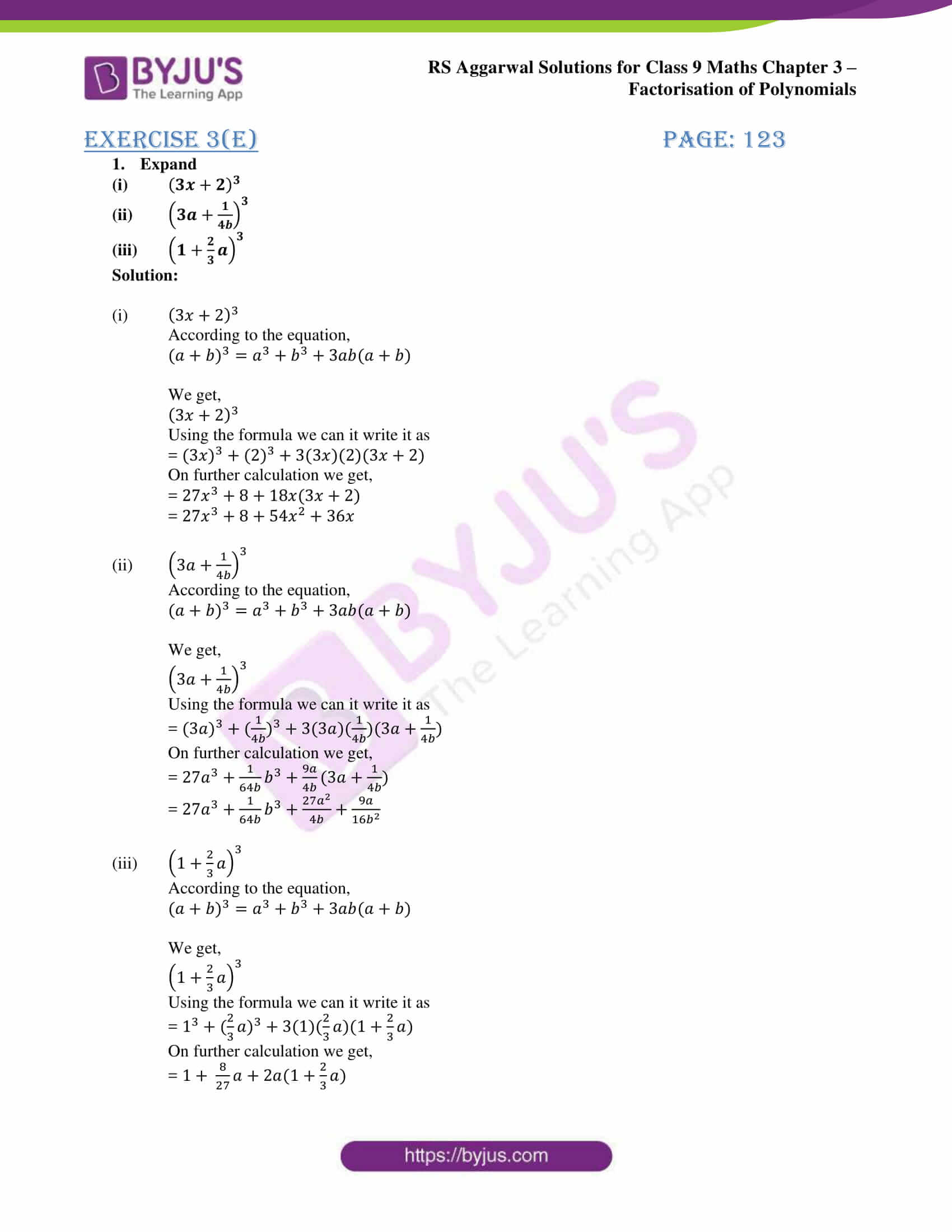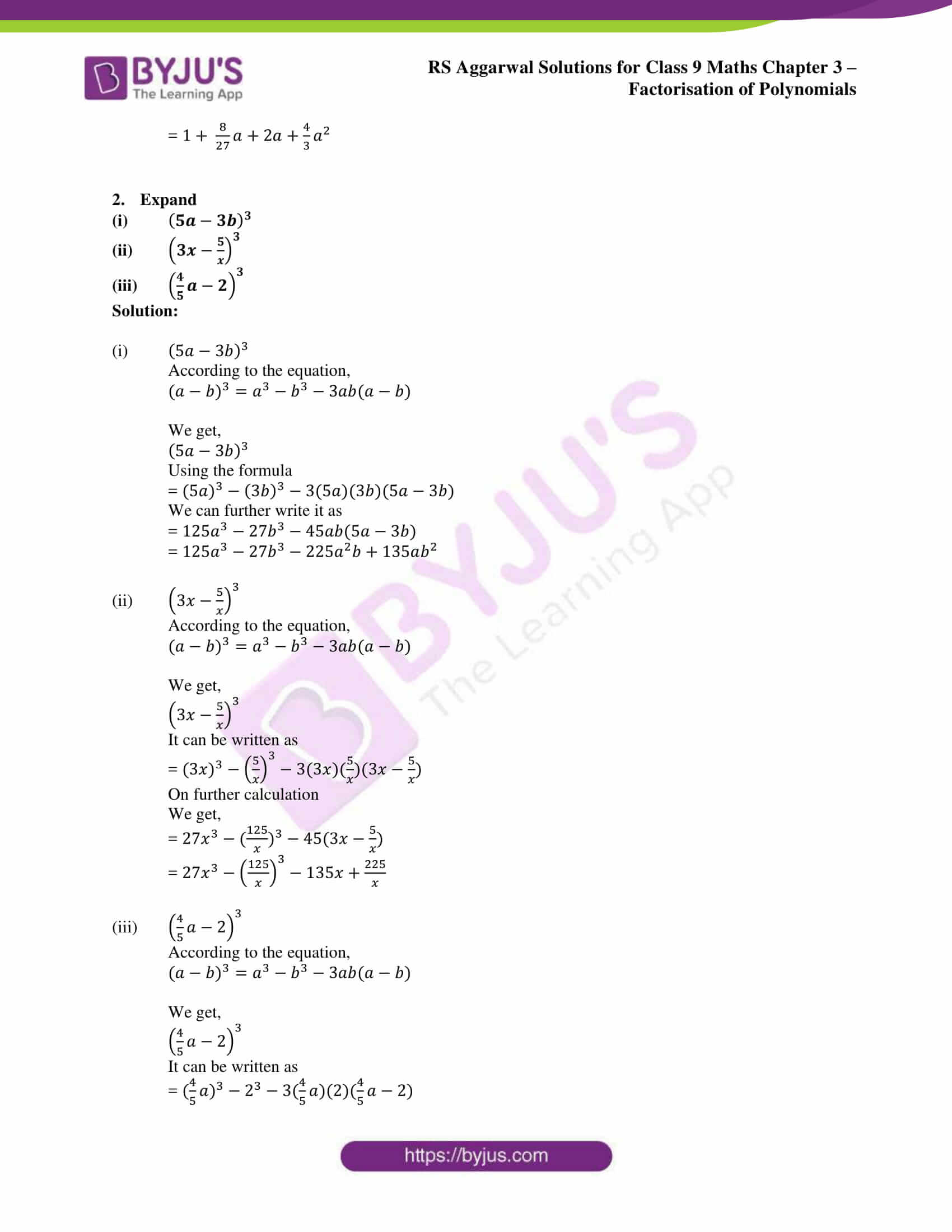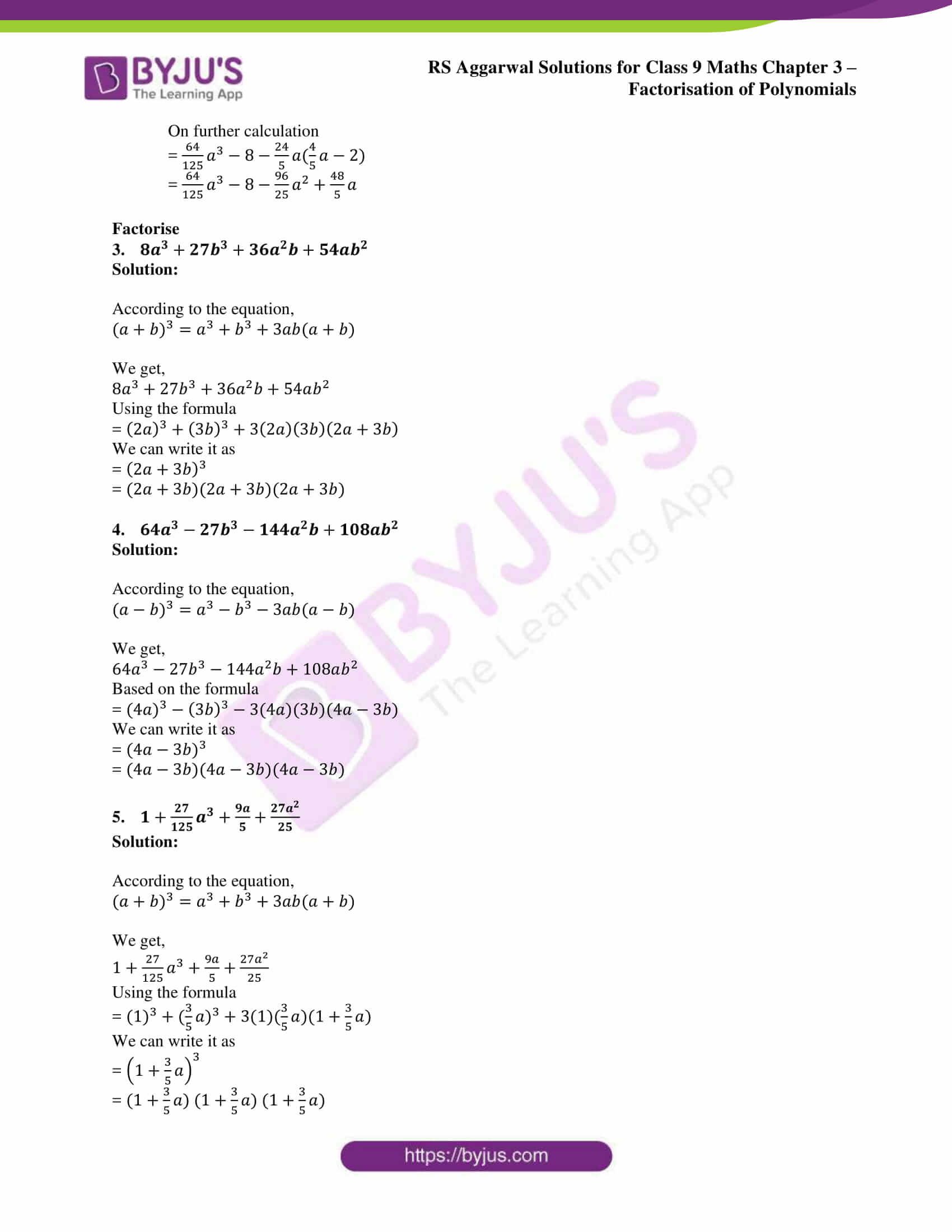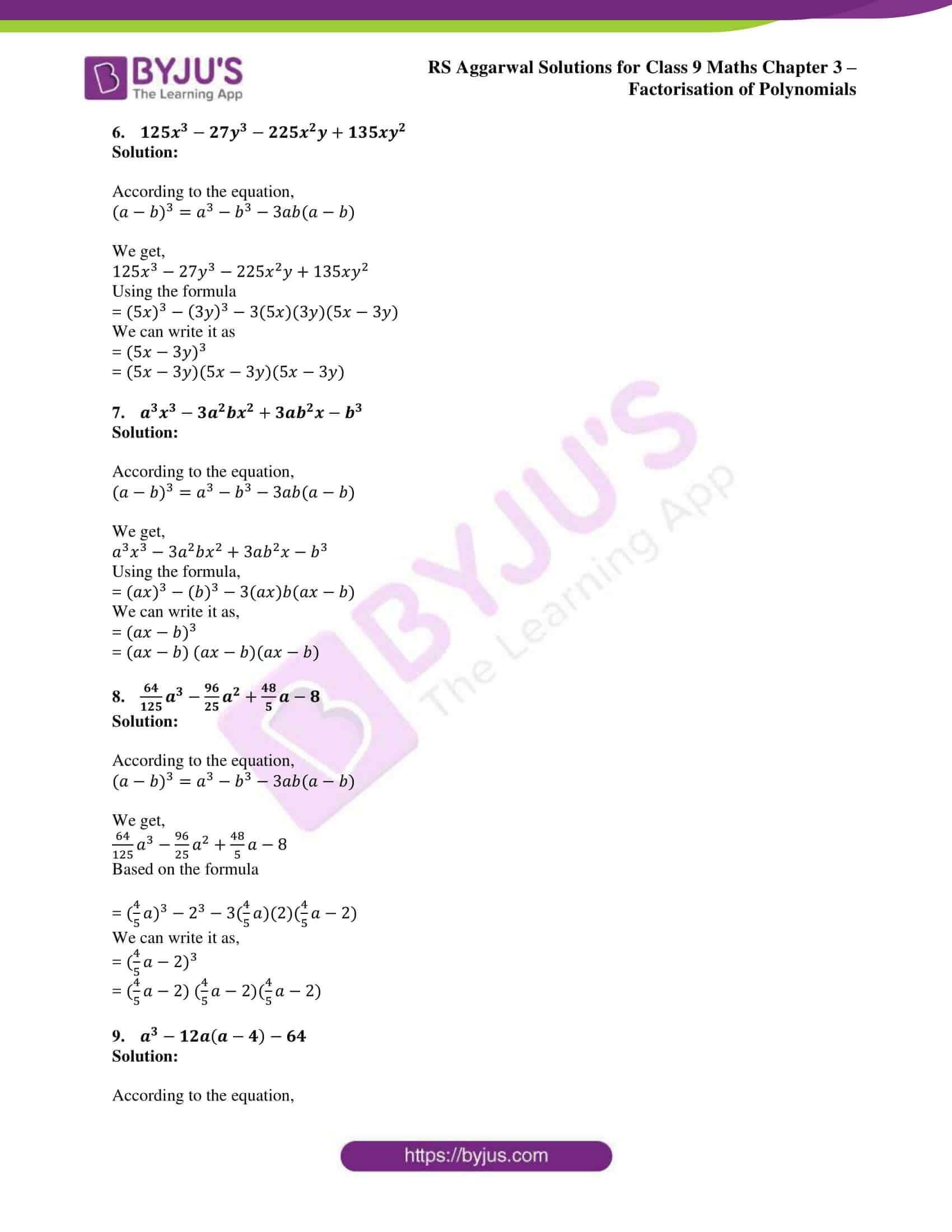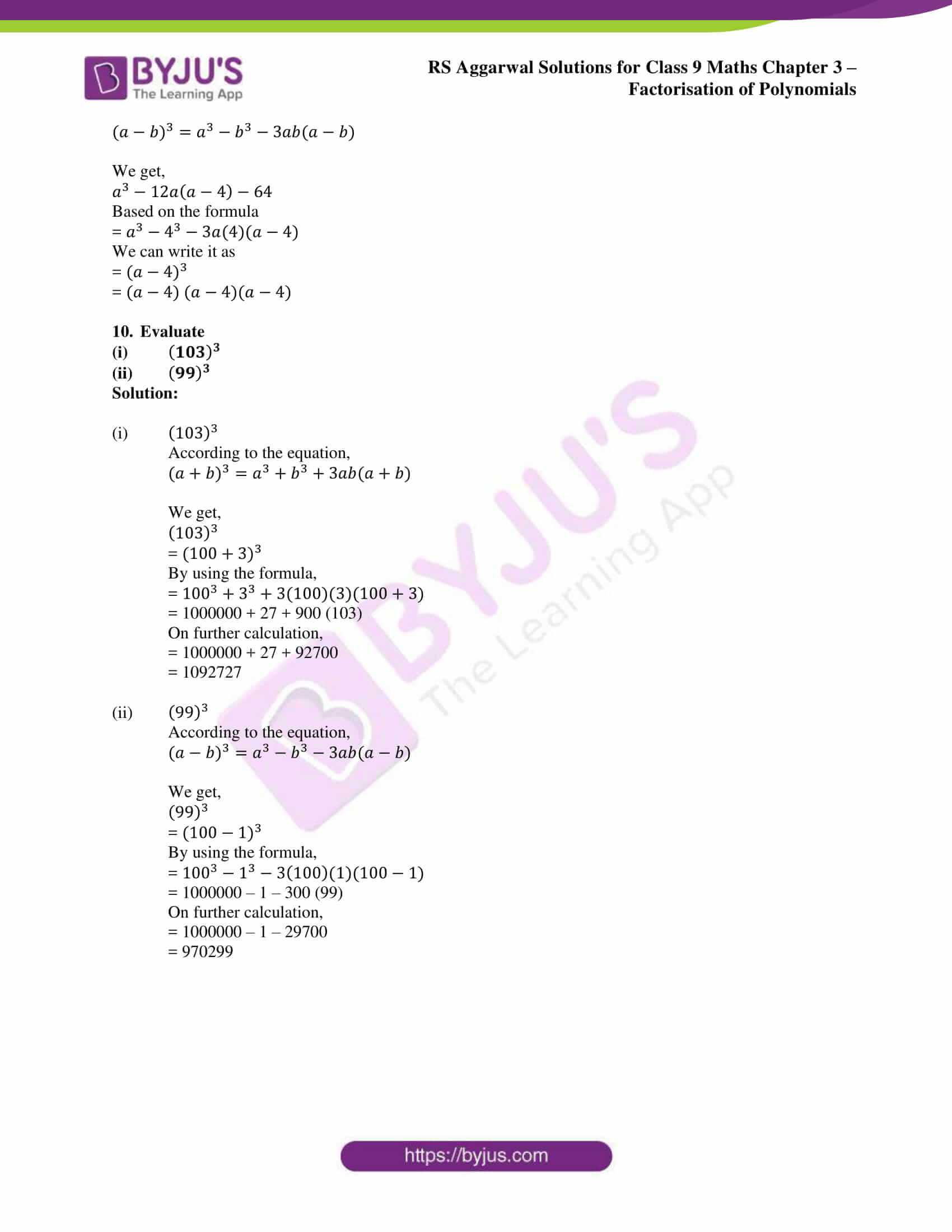### Access other exercise solutions of Class 9 Maths Chapter 3: Factorisation of Polynomials

Exercise 3A Solutions 34 Questions

Exercise 3B Solutions 40 Questions

Exercise 3C Solutions 66 Questions

Exercise 3D Solutions 7 Questions

Exercise 3F Solutions 38 Questions

Exercise 3G Solutions 25 Questions

### RS Aggarwal Solutions Class 9 Maths Chapter 3 – Factorisation of Polynomials Exercise 3E

RS Aggarwal Solutions Class 9 Maths Chapter 3 Factorisation of Polynomials Exercise 3E has examples and solutions in a descriptive manner for the fifth exercise to find the cube of a binomial.

### Key features of RS Aggarwal Solutions for Class 9 Maths Chapter 3: Factorisation of Polynomials Exercise 3E

• The revision of Exercise 3E in the third chapter can be done in less time by using the RS Aggarwal Solutions for Class 9.
• The students by solving problems on a daily basis can recognize their weakness and work on it to score well.
• The primary aim of creating RS Aggarwal solutions is to help students have easy access to the answers for scoring better.
• Our experts prepare the solutions keeping in mind that it should be easy for the students to understand concepts and grasp it faster.